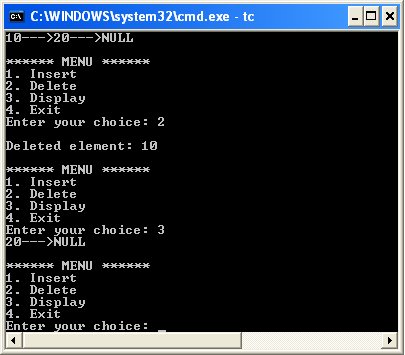The perfect place for easy learning...

# Data Structures

×

## Topics List

The major problem with the queue implemented using an array is, It will work for an only fixed number of data values. That means, the amount of data must be specified at the beginning itself. Queue using an array is not suitable when we don't know the size of data which we are going to use. A queue data structure can be implemented using a linked list data structure. The queue which is implemented using a linked list can work for an unlimited number of values. That means, queue using linked list can work for the variable size of data (No need to fix the size at the beginning of the implementation). The Queue implemented using linked list can organize as many data values as we want.

In linked list implementation of a queue, the last inserted node is always pointed by 'rear' and the first node is always pointed by 'front'.

# ExampleIn above example, the last inserted node is 50 and it is pointed by 'rear' and the first inserted node is 10 and it is pointed by 'front'. The order of elements inserted is 10, 15, 22 and 50.

# Operations

To implement queue using linked list, we need to set the following things before implementing actual operations.

• Step 1 - Include all the header files which are used in the program. And declare all the user defined functions.
• Step 2 - Define a 'Node' structure with two members data and next.
• Step 3 - Define two Node pointers 'front' and 'rear' and set both to NULL.
• Step 4 - Implement the main method by displaying Menu of list of operations and make suitable function calls in the main method to perform user selected operation.

# enQueue(value) - Inserting an element into the Queue

We can use the following steps to insert a new node into the queue...

• Step 1 - Create a newNode with given value and set 'newNode → next' to NULL.
• Step 2 - Check whether queue is Empty (rear == NULL)
• Step 3 - If it is Empty then, set front = newNode and rear = newNode.
• Step 4 - If it is Not Empty then, set rear → next = newNode and rear = newNode.

# deQueue() - Deleting an Element from Queue

We can use the following steps to delete a node from the queue...

• Step 1 - Check whether queue is Empty (front == NULL).
• Step 2 - If it is Empty, then display "Queue is Empty!!! Deletion is not possible!!!" and terminate from the function
• Step 3 - If it is Not Empty then, define a Node pointer 'temp' and set it to 'front'.
• Step 4 - Then set 'front = front → next' and delete 'temp' (free(temp)).

# display() - Displaying the elements of Queue

We can use the following steps to display the elements (nodes) of a queue...

• Step 1 - Check whether queue is Empty (front == NULL).
• Step 2 - If it is Empty then, display 'Queue is Empty!!!' and terminate the function.
• Step 3 - If it is Not Empty then, define a Node pointer 'temp' and initialize with front.
• Step 4 - Display 'temp → data --->' and move it to the next node. Repeat the same until 'temp' reaches to 'rear' (temp → next != NULL).
• Step 5 - Finally! Display 'temp → data ---> NULL'.

# Implementation of Queue Datastructure using Linked List - C Programming

``````#include<stdio.h>
#include<conio.h>

struct Node
{
int data;
struct Node *next;
}*front = NULL,*rear = NULL;

void insert(int);
void delete();
void display();

void main()
{
int choice, value;
clrscr();
printf("\n:: Queue Implementation using Linked List ::\n");
while(1){
printf("1. Insert\n2. Delete\n3. Display\n4. Exit\n");
scanf("%d",&choice);
switch(choice){
case 1: printf("Enter the value to be insert: ");
scanf("%d", &value);
insert(value);
break;
case 2: delete(); break;
case 3: display(); break;
case 4: exit(0);
default: printf("\nWrong selection!!! Please try again!!!\n");
}
}
}
void insert(int value)
{
struct Node *newNode;
newNode = (struct Node*)malloc(sizeof(struct Node));
newNode->data = value;
newNode -> next = NULL;
if(front == NULL)
front = rear = newNode;
else{
rear -> next = newNode;
rear = newNode;
}
printf("\nInsertion is Success!!!\n");
}
void delete()
{
if(front == NULL)
printf("\nQueue is Empty!!!\n");
else{
struct Node *temp = front;
front = front -> next;
printf("\nDeleted element: %d\n", temp->data);
free(temp);
}
}
void display()
{
if(front == NULL)
printf("\nQueue is Empty!!!\n");
else{
struct Node *temp = front;
while(temp->next != NULL){
printf("%d--->",temp->data);
temp = temp -> next;
}
printf("%d--->NULL\n",temp->data);
}
}```
```

Output